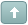Welcome Guest! You need to login or register to make posts.Error

# HOWTO: Reset Rotation of VObjects

 Tamila #1 Posted : Sunday, March 15, 2009 8:03:58 PM(UTC)Rank: Advanced MemberGroups: Joined: 3/9/2008(UTC)Posts: 554Was thanked: 1 time(s) in 1 post(s) I am using Vector Objects in my project. After VObject was roteted I want to reset this rotate angle back to 0.The following code allows to calculate the angle between horizon and VObject using Transformation matrix. After you get the angle you can transform VObject matrix and back VObject angle to 0.Code:``````' ' Reset Angle button ' Private Sub _resetButton_Click(ByVal sender As System.Object, ByVal e As System.EventArgs) Handles _resetButton.Click If _mlv.CurrentDesigner.VObjects.Length > 0 Then Dim obj As VObject = _mlv.CurrentDesigner.VObjects(0) ResetObjectAngle(obj) End If End Sub ' ' Reset Angle function ' Private Sub ResetObjectAngle(ByVal obj As VObject) Dim angle As Single = GetVObjectAngle(obj) Dim bounds As RectangleF = obj.GetTransformedVObjectBounds() Dim point As New PointF(bounds.Left + bounds.Width / 2, bounds.Top + bounds.Height / 2) If angle <> 0 Then obj.Transform.RotateAt(-angle, point, Drawing2D.MatrixOrder.Append) obj.Update() End If End Sub Private Shared Function GetVObjectAngle(ByRef obj As VObject) As Single Dim skyline As PointF = New PointF(1000, 0) Dim objSkyline(1) As PointF objSkyline(0) = New PointF(1000, 0) obj.Transform.TransformVectors(objSkyline) Return CalcAngle(skyline, objSkyline(0)) End Function ' ' Calculates angle between 2 specified vectors. ' Private Shared Function CalcAngle( _ ByVal v0 As System.Drawing.PointF, _ ByVal v1 As System.Drawing.PointF) As Single Dim dotProduct As Single = v0.X * v1.X + v0.Y + v1.Y Dim vLengthProduct As Single = CalcLength(v0) * CalcLength(v1) If vLengthProduct < 0.01 Then Throw New ApplicationException("Ups, div by zero error...") End If Dim result As Single = _ CType(Math.Acos(dotProduct / vLengthProduct) / Math.PI * 180, Single) Dim vecProduct As Single = v0.X * v1.Y - v0.Y * v1.X If vecProduct < 0 Then result = 360 - result End If Return result End Function ' ' Calculates length of the specified vector. ' Private Shared Function CalcLength(ByVal v0 As System.Drawing.PointF) As Single Return CType(Math.Sqrt(v0.X * v0.X + v0.Y * v0.Y), Single) End Function `````` Edited by moderator Monday, May 28, 2012 8:32:56 PM(UTC)  | Reason: Not specifiedUsers browsing this topic
Forum Jump
You cannot post new topics in this forum.
You cannot reply to topics in this forum.
You cannot delete your posts in this forum.
You cannot edit your posts in this forum.
You cannot create polls in this forum.
You cannot vote in polls in this forum.